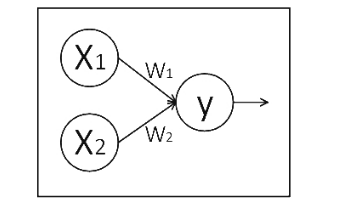TensorFlow基础知识

## 1 综述

TensorFlow的编程系统中：

1. 使用张量(`tensor`)来表示数据
2. 使用图(`graph`)来表示计算任务。 图中的节点被称之为 op (operation 的缩写). 一个 op 获得 0 个或多个 Tensor, 执行计算, 产生 0 个或多个 Tensor. 每个 Tensor 是一个类型化的多维数组。
3. 使用会话(`Session`)的上下文(`context`)中执行图
4. 通过变量(`Variable`)维护状态
5. 使用 `feed``fetch` 赋值和获取数据.

## 2 张量(Tensor)

• 0阶张量称作标量(scalar),表示一个单独的数;举例 S=123
• 1阶张量称作向量(vector),表示一个一维数组;举例 V=[1,2,3]
• 2阶张量称作矩阵(matrix),表示一个二维数组,它可以有 i 行 j 列个元素,每个元素可
以用行号和列号共同索引到;
举例 m=[[1, 2, 3], [4, 5, 6], [7, 8, 9]]

``````import tensorflow as tf
a=tf.constant([1.0,2.0])
b=tf.constant([3.0,4.0])
result=a+b
print(result)``````

``````Tensor("add:0", shape=(2,), dtype=float32)
``````

• shape :维度信息，括号里只有一个数“2“，表示维度是1且一个维度里有两个元素
• dtpye :数据类型

``````c=tf.constant([1.0,2.0])  #Tensor("Const_2:0", shape=(2,), dtype=float32) 是个向量，有两个元素
d=tf.constant([[1.0,2.0]])  #Tensor("Const_3:0", shape=(1, 2), dtype=float32) 1行2列矩阵
e=tf.constant([[1.0],[2.0]])  #Tensor("Const_4:0", shape=(2, 1), dtype=float32) 2行1列矩阵
``````

## 2 计算图x1、x2 表示输入,w1、w2 分别是 x1 到 y 和 x2 到 y 的权重,y=x1w1+x2w2。

``````import tensorflow as tf #引入模块
x = tf.constant([[1.0, 2.0]]) #定义一个 2 阶张量等于[[1.0,2.0]]
w = tf.constant([[3.0], [4.0]]) #定义一个 2 阶张量等于[[3.0],[4.0]]
y = tf.matmul(x, w) #实现 xw 矩阵乘法
print y #打印出结果
``````

``````Tensor(“matmul:0”, shape(1,1), dtype=float32),
``````

## 3 会话

``````with tf.Session() as sess:
print(sess.run(y))
``````

【举例】

``````import tensorflow as tf #引入模块
x = tf.constant([[1.0, 2.0]]) #定义一个 2 阶张量等于[[1.0,2.0]]
w = tf.constant([[3.0], [4.0]]) #定义一个 2 阶张量等于[[3.0],[4.0]]
y = tf.matmul(x, w) #实现 xw 矩阵乘法
print y #打印出结果
with tf.Session() as sess:
print(sess.run(y)) #执行会话并打印出执行后的结果``````

``````Tensor(“matmul:0”, shape(1,1), dtype=float32)
[[11.]]
``````

## 4 变量

### 4.1 变量创建

``````# 创建一个变量, 初始化为标量 0.
state = tf.Variable(0, name="counter")

# 权重和偏置
weights = tf.Variable(tf.random_normal([784, 200], stddev=0.35),name="weights")
biases = tf.Variable(tf.zeros(), name="biases")
``````

``````tf.random_normal()   #生成正态分布随机数
tf.truncated_normal() #生成去掉过大偏离点的正态分布随机数
tf.random_uniform()  #生成均匀分布随机数
tf.zeros         #表示生成全 0 数组
tf.ones       #表示生成全 1 数组
tf.fill     #表示生成全定值数组
tf.constant #表示生成直接给定值的数组``````

### 4.2 变量初始化

#### 4.2.1 tf.initialize_all_variables()

``````# Create two variables.
weights = tf.Variable(tf.random_normal([784, 200], stddev=0.35),
name="weights")
biases = tf.Variable(tf.zeros(), name="biases")
...
# Add an op to initialize the variables.
init_op = tf.initialize_all_variables()

# Later, when launching the model
with tf.Session() as sess:
# Run the init operation.
sess.run(init_op)
...
# Use the model
...``````

#### 4.2.2 initialized_value()

。由于tf.initialize_all_variables()是并行地初始化所有变量，有时候会需要用另一个变量的初始化值给当前变量初始化。

``````# Create a variable with a random value.
weights = tf.Variable(tf.random_normal([784, 200], stddev=0.35),
name="weights")
# Create another variable with the same value as 'weights'.
w2 = tf.Variable(weights.initialized_value(), name="w2")
# Create another variable with twice the value of 'weights'
w_twice = tf.Variable(weights.initialized_value() * 0.2, name="w_twice")
``````

### 4.3 保存和加载

#### 4.3.1 保存变量

``````# Create some variables.
v1 = tf.Variable(..., name="v1")
v2 = tf.Variable(..., name="v2")
...
# Add an op to initialize the variables.
init_op = tf.initialize_all_variables()

# Add ops to save and restore all the variables.
saver = tf.train.Saver()

# Later, launch the model, initialize the variables, do some work, save the
# variables to disk.
with tf.Session() as sess:
sess.run(init_op)
# Do some work with the model.
..
# Save the variables to disk.
save_path = saver.save(sess, "/tmp/model.ckpt")
print "Model saved in file: ", save_path``````

#### 4.3.2 恢复变量

``````# Create some variables.
v1 = tf.Variable(..., name="v1")
v2 = tf.Variable(..., name="v2")
...
# Add ops to save and restore all the variables.
saver = tf.train.Saver()

# Later, launch the model, use the saver to restore variables from disk, and
# do some work with the model.
with tf.Session() as sess:
# Restore variables from disk.
saver.restore(sess, "/tmp/model.ckpt")
print "Model restored."
# Do some work with the model
...``````

## 5 Fetch和Feed

### 5.1 Fetch

``````# 创建一个变量, 初始化为标量 0.
state = tf.Variable(0, name="counter")

# 创建一个 op, 其作用是使 state 增加 1

one = tf.constant(1)
update = tf.assign(state, new_value)

# 启动图后, 变量必须先经过`初始化` (init) op 初始化,
# 首先必须增加一个`初始化` op 到图中.
init_op = tf.initialize_all_variables()

# 启动图, 运行 op
with tf.Session() as sess:
# 运行 'init' op
sess.run(init_op)
# 打印 'state' 的初始值
print sess.run(state)
# 运行 op, 更新 'state', 并打印 'state'
for _ in range(3):
sess.run(update)
print sess.run(state)

# 输出:

# 0
# 1
# 2
# 3``````

``````input1 = tf.constant(3.0)
input2 = tf.constant(2.0)
input3 = tf.constant(5.0)
mul = tf.mul(input1, intermed)

with tf.Session():
result = sess.run([mul, intermed])
print result

# 输出:
# [array([ 21.], dtype=float32), array([ 7.], dtype=float32)]``````

### 5.2 Feed

feed 使用一个 tensor 值临时替换一个操作的输出结果. 你可以提供 feed 数据作为 run() 调用的参数. feed 只在调用它的方法内有效, 方法结束, feed 就会消失. 最常见的用例是将某些特殊的操作指定为 “feed” 操作, 标记的方法是使用 tf.placeholder() 为这些操作创建占位符.

``````input1 = tf.placeholder(tf.types.float32)
input2 = tf.placeholder(tf.types.float32)
output = tf.mul(input1, input2)

with tf.Session() as sess:
print sess.run([output], feed_dict={input1:[7.], input2:[2.]})

# 输出:
# [array([ 14.], dtype=float32)]
``````

目录